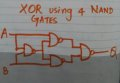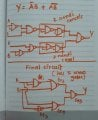# How to employ XOR Gate using just 4 NAND gates?

#### Devika B S

Joined Mar 8, 2017
144
According to many sources (for instance Wikipedia), it's possible to obtain XOR gate using 4 NAND gates.
However, when I try to do so step by step (by replacing all gates using NAND and cancelling two NAND gates that are in cascade), I always end up getting 5 NAND gates instead of 4. Is there a step by step procedure by which XOR gates are implemented using just 4 NAND gates?

XOR using 4 NANDReduction which I used and the resulting circuit#### MrAl

Joined Jun 17, 2014
6,500
Hi,

Start by looking at the various ways to express an XOR gate as there are several different ways.
A good starting point is:
c=(b+a)*(bn+an)

[an=a' and bn=b']

and i think that is a good place to start because the NAND gate is c=(an+bn) which expresses it as a negative logic nor gate and where you can start to see the simplicity that we get when using Boolean logic simply because there are only two states.

There are ideas from automated reasoning that help to reduce logic circuits but you'd have to read up on that.

Last edited:

#### Devika B S

Joined Mar 8, 2017
144
Hi,

Start by looking at the various ways to express an XOR gate as there are several different ways.
A good starting point is:
c=(b+a)*(bn+an)

[an=a' and bn=b']

and i think that is a good place to start because the NAND gate is c=(an+bn) which expresses it as a negative logic nor gate and where you can start to see the simplicity that we get when using Boolean logic simply because there are only two states.

There are ideas from automated reasoning that help to reduce logic circuits but you'd have to read up on that.
I thought that K-Map gives the maximum possible reduction in the number of combinations involved. I am surprised that it does not work while converting all gates to NAND gates.

#### WBahn

Joined Mar 31, 2012
24,700
According to many sources (for instance Wikipedia), it's possible to obtain XOR gate using 4 NAND gates.
However, when I try to do so step by step (by replacing all gates using NAND and cancelling two NAND gates that are in cascade), I always end up getting 5 NAND gates instead of 4. Is there a step by step procedure by which XOR gates are implemented using just 4 NAND gates?

XOR using 4 NAND
View attachment 134488

The four-NAND XOR uses a pretty clever trick to sneak two signals out of one.

The easiest way to see it is to start with the four-NAND layout and convert it to two ANDs and two ORs (with two additional inverters feeding the first OR).

Looking at the two AND gates and the final OR gate, you see that you have the XOR logic, complete with the non-inverted input signals going into the two AND gates. Now all you need is to get the complemented signals from the other input into each gate. Normally that would require two inverters to generate two signals and all you have left to it with is a single NAND gate that can only produce one signal.

But what if you could mix the two signals in such a way that that the respective AND gates filtered out the one you don't want and let the one you do want pass through?

Y = A·B' + A'·B

What you need is

Y = A·X + X·B

where X is a function of A and B. So you need

A·X = A·B'
and
X·B = A'·B

This would SEEM to indicate that X has to simultaneously be A' and B' -- two signals at the same time. So let's make it both at the same time. X = A' + B'. When we AND A and X, the A kills the A' terms, while when we AND B and X, B kills the B' term.

With all of that in mind, see if you can now start with

Y = A·B' + A'·B

Y = { [A · (A·B)']' · [(A·B)' · B]' }'

It can be done in about four steps.

•Devika B S

#### WBahn

Joined Mar 31, 2012
24,700
I thought that K-Map gives the maximum possible reduction in the number of combinations involved. I am surprised that it does not work while converting all gates to NAND gates.
•